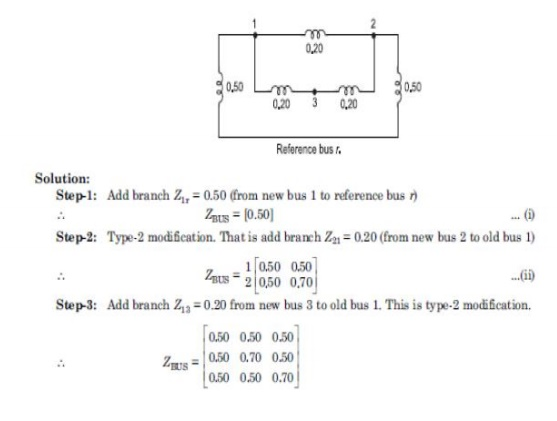Home | | Power System Analysis | Solved problems: Fault Analysis - Balanced Faults

# Solved problems: Fault Analysis - Balanced Faults

Power System Analysis - Fault Analysis - Balanced Faults - Solved problems: Fault Analysis - Balanced Faults

SOLVED PROBLEMS

Problem 1

A synchronous generator and a synchronous motor each rated 20MVA, 12.66KV having 15% reactance are connected through transformers and a line as shown in fig. the transformers are rated 20MVA,12.66/66KV and 66/12.66KV with leakage reactance of 10% each. The line has a reactance of 8% on base of 20MVA, 66 KV. The motor is drawing 10MW at 0.8 leading power factors and a terminal voltage 11KV when symmetrical three phase fault occurs at the motors terminals. Determine the generator and motor currents. Also determine the fault current.Two generators G1 and G2 are rated 15MVA, 11KV and 10MVA, 11KV respectively. The generators are connected to a transformer as shown in fig. Calculate the sub transient current in each generator when a three phase fault occurs on the high voltage side of the transformer.A radial power system network is shown in fig. a three phase balanced fault occurs at F. Determine the fault current and the line voltage at 11.8 KV bus under fault condition.Problem : 2

A 100MVA,11KV generator with X’’=0.20 p.u is connected through a transformer and line to a bus bar that supplies three identical motor as shown in fig. and each motor has X’’=0.20 p.u and X’=0.25 p.u on a base of 20MVA,33KV.the bus voltage at the motors is 33KV when a three phase balanced fault occurs at the point F. Calculate

(a)        subtransient current in the fault

(b)       subtransient current in the circuit breaker B

(c)        Momentary current in the circuit breaker B

(d)       The current to be interrupted by CB B in (i) 2 cycles (ii) 3 cycles (iii) 5 cycles (iv) 8 cyclesObtain impedance matrix ZBUS for shown in figure.Study Material, Lecturing Notes, Assignment, Reference, Wiki description explanation, brief detail
Power System Analysis : Fault Analysis : Balanced Faults : Solved problems: Fault Analysis - Balanced Faults |